# SOLVESYS 49 version 1.2

## Numerical Nonlinear Equations and Least Squares Solver  for the HP49G calculator

### DISCLAIMER

SOLVESYS.LIB AND THIS MANUAL ARE PRESENTED WITHOUT WARRANTIES, EXPRESSED OR IMPLIED. THE AUTHOR MAKES NO GUARANTEE AS TO THE FITNESS OF THIS SOFTWARE.

SOLVESYS.LIB CAN BE COPIED FREELY BUT MAY NOT BE USED FOR COMMERCIAL PURPOSES WITHOUT PERMISSION FROM THE AUTHOR.

### OVERVIEW

This documentation covers SOLVESYS 1.2 for the HP49G. Latest version of the software is downloadable from http://solvesys.cjb.net. Also a FAQ is available from here if you have any questions not covered in this manual.

SOLVESYS 49 requires an HP49G with ROM 1.10 or later. It will not work properly with the ROM 1.05 shipped in early versions of the calculator.

### INSTALLATION

SOLVESYS is a library and must be properly installed in order to work  Consult the Advanced User's Guide (section 11-6) for details on this. However, assuming RPN mode, the following should work:

1. Transfer SOLVESYS.LIB to the HP49G - use binary transfer.
2. Recall the library to the stack and press n [STO], where n is the desired port (0, 1 or 2). After storing the library, you can purge the original copy to save memory.
3. Press [ON]+[C] to restart the HP49G. This completes the installation.

To verify that this library has been correctly installed, press [CAT], find the command ABOUTSS and press [ENTER]. The version number displayed should be "SOLVESYS 49 1.2" (the current build is 17/07/00).

SOLVESYS can now be started in several ways, for example:

• Using the NUM.SLV menu (SOLVESYS 1.2 appears as the last item)
• Using CAT (command is listed as SOLVESYS).

To remove the SOLVESYS library, do this:

1. Start the FILE MANAGER
2. Select the port (0, 1 or 2) where SOLVESYS is installed and press [OK]
3. Press [NXT] and [PURGE].

If calculator returns an "Object in use" error at this point, press [ON]+[C] and repeat the above steps.

To transfer SOLVESYS.LIB to another HP49G.:

1. Put the library ID (n: 1550) in the stack and press [RCL].
2. Store the returned library in any variable
3. Transfer this variable to the other calc. - use binary transfer.

### WHAT IS SOLVESYS?

SOLVESYS 49 is an environment for solving systems of linear and nonlinear equations. It is also capable of doing nonlinear least-squares minimization.

To be more precise, SOLVESYS is designed to either zero or least-squares minimize m linear or nonlinear equations in n unknowns.When m=n, the problem is obviously to find a solution satisfying all equations. If m>n, the equations are generally inconsistent so instead SOLVESYS searches for a least squares solution, i.e. the minimizer of the sum of squared equation residuals. Least squares minimization is commonly used in datafitting applications. The case n>m is perhaps of less interest but is also handled by SOLVESYS.

Note that SOLVESYS is a numeric solver -  it will try to solve the equations using iterative methods, i.e. the user provides some initial guess or estimate of the solution which the solver (hopefully) can use to find the true solution. It can be necessary to try out different starting guesses before the solver succeeds in this quest, but if good starting values are provided, equations can often be solved with only a few iterations.

SOLVESYS does not allow units in equations or variables (too slow), also it cannot be used to solve systems with discrete functions or variables.

The solver engine is based on a (Gauss-) Newton method with a mixed quadratic and cubic linesearch procedure. For more details on this algorithm, see Dennis and Schnabel  or Press et al. .

 Dennis, J.E., and Schnabel, R.B. 1983, Numerical Methods for Unconstrained Optimization and Nonlinear Equations (Englewood Cliffs, NJ: Prentice-Hall).
 Press, W.H. et al. 1992, Numerical Recipes in C: The Art of Scientific Computing. 2nd ed. (Cambridge: University Press).

Rather than describing each screen and menu in SOLVESYS 49, I'll provide some examples instead.

Basic usage is described in the first example so please start from here even though you may not be interested in solving linear equations.

Example 1: Solving a system of linear equations

Although the HP49G already has built-in functions to solve systems of linear equations (such as LINSOLVE), you may find it convenient to use SOLVESYS instead. At least this small example should give you an idea of how to use SOLVESYS.

The system we want to solve is:

1. 2x + y = 3x + z

2. z + x = 2(x + y)

3. (z + x)/2 + y + 1.2 = 0

There are several ways to start SOLVESYS. The fastest way is open the NUM.SLV choose menu and select SOLVESYS 1.2 from here.

The first thing SOLVESYS displays, is a list of equations to be solved. This is actually the contents of the 'EQ' variable so if there's no 'EQ' variable in the current directory an empty list is displayed. For example, if the above equations are stored as a list in 'EQ' prior to starting SOLVESYS, the opening display should look something like this: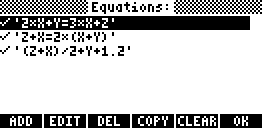If you haven't stored the equations in 'EQ', use [ADD] to enter the equations.

The checkmark indicates that the equation is marked for solving (default). If there are equations you don't want to solve, use the [+/-] key to uncheck them - this has the same effect as [DEL] but does not remove the equation from 'EQ'.

Now press [OK] to proceed. SOLVESYS analyzes the equations for variables and opens the "Variables" screen shown below.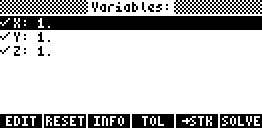You can modify a highlighted value using the [EDIT] menukey or [ENTER]. To check/uncheck a variable, use the [+/-] key. The [RESET] key sets all variables equal to 1.

• Checked variables will be solved for (default). A real or complex valued starting guess must be provided. This should be your best guess of the solution you're looking for - don't use the default values if you know any better.
• Unchecked variables are not solved for and the value remain constant during the solving process. In fact, the value need not be a real or complex numbers, however the equations must eventually evaluate to a number.

In this example, we want to solve for all variables so all must be checked. The starting values are unimportant because the equations are linear. If we use the default values as shown and press [SOLVE] we get the next screens: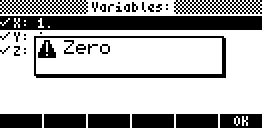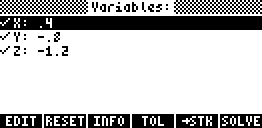Here "Zero" indicates that a solution has been found. When returned to the "Variables" screen, the final values are displayed.

As you can see, the solution is (x, y, z) = (0.4, -0.8, -1.2). When you exit SOLVESYS, these values will be stored in their respective variables. However you can also use [->STK] to copy this solution (as well as a error vector) to the stack

Example 2: Solving a system of nonlinear equations.

In this example we will solve a rather large system (propane combustion?) which is a mixture of 11 linear and nonlinear equations in 11 unknowns (x1,...,x11) and seven known variables (a1,...,a6 and r).

 x1 + x4 = 3 2x1 + x2 + x4 + x7 + x8 + x9 + 2x10 = 10 + r x2 + 2x5 + x6 + x7 = 8 2x3 + x5 = 4r x1 x5 = a1 x2 x4 x6 x2½ = a2 (x2 x4 x11)½ x7 x4½ = a3 (x1 x4 x11)½ x8 x4 = a4 x2 x11 x9 x4 = a5 x1 (x3 x11)½ x10 x42 = a6 x42 x11 x11 = x1 + x2 + x3 + x4 + x5 + x6 + x7 + x8 + x9 + x10 a1 = 0.193 a2 = 0.002597 a3 = 0.003448 a4 = 0.00001799 a5 = 0.0002155 a6 = 0.00003846 r = 4.056734

In SOLVESYS, this equation set will look like: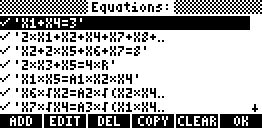All the equations should be included (checked). Press [OK] to open the "Variables" screen: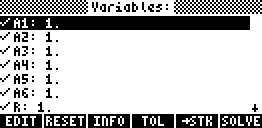Remember that the first seven values here are constants. These must be unchecked and filled in with their corresponding values shown earlier. For the unknowns we will use 1 as the initial value except x11 we set to 10 since x11 is the sum of the other variables (equation 11).

When finished, the display should then look something like the one below. Use the downarrow key to view/edit the remaining variables.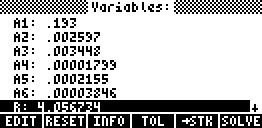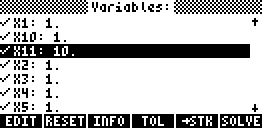[Note: To verify that you have the correct amount of unknowns, press [INFO] menukey. This should show "m/n = 11/11" which means that 11 equations and 11 variables are selected to be solved]

When done, press [SOLVE]and after a few minutes a solution (Zero) is found: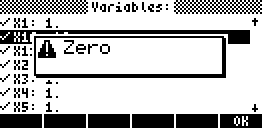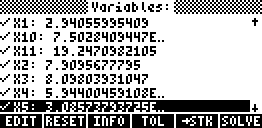The final values can be viewed using the up/downarrow keys. [->STK] can be used to copy the result and the equation residuals the stack (do this!). Note that the values are also stored in the variables after you exit SOLVESYS.

Example 3: Nonlinear least-squares

If there are more (generally inconsistent) equations than unknowns, SOLVESYS will search for a "best fit" solution in the least-squares sense, ie. a solution that minimizes the sum of squared equations deviations.

A common application of least-squares minimization is datafitting, where one is attempting to fit m observations to an expression of n unknown parameters for which the "best-fit" values are required. If each observation is inserted in the expression, the result is a system of m "observational" equations in n unknowns.

Here's an example.

The relationship between the measured pressure and temperature in saturated steam can be written as

Y=a*ALOG(b*T/(c+T))

where Y is the measured pressure of the steam at various values of a controllable temperature T
a, b and c are unknown parameters (to be estimated)

The following data were collected.

 T 0 10 20 30 40 50 60 70 80 85 90 95 100 105 Y 4.14 8.52 16.31 32.18 64.62 98.76 151.13 224.74 341.35 423.36 522.78 674.32 782.04 920.01

To create the 14 equations needed, perform the following four steps:

1. Store the data as lists in variables such as 'T' and 'Y'.
2. If any of the variables A, B or C exist, they must be purged.
3. Enter 'Y=A*ALOG(B*T/(C ADD T))'on the stack and press [EVAL]
4. You're done! Store the resulting list of equations in the 'EQ' variable.

[IMPORTANT: In step 3. you must replace any occurence of "+" with "ADD" or "--"  since "+" has a different action when list are involved.]

As for the initial guesses, note that the first equation is actually A=4.14 so this can be used as a guess. If A=4.14 is inserted in the next two equations, these can be reduced to two linear equations with the solution (b,c) = (5.93,179). Thus an initial guess could be (a,b,c)=(4,6,180).

Now we're ready to start SOLVESYS.  The equations are already created so press [OK] and fill in the guess values (a,b,c) = (4, 6, 180)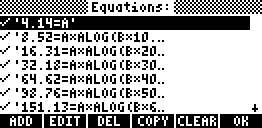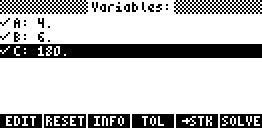Press [SOLVE] and answer "yes" to accept that m is different from n (since we have more equations than unknowns). After a few iterations a solution (minimum) is found.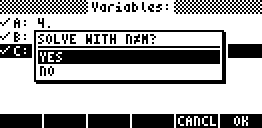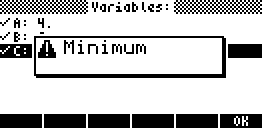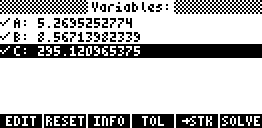Using 3 significant digits, the best fit function is Y(T)=5.27*ALOG[8.56*T/(295+T)].

Be aware that there are no simple ways to distinguish between a local or global minimum so the choice of starting values is very crucial. A good initial guess is not only a real time-saver, it will also pick out the right (global) minimum for you!

### ERROR TOLERANCES

It is possible to modify the error tolerances used for convergence testing although it is not recommended. Use [TOL] to change any the error tolerances described below.

XTOL

Tolerance for the convergence of iterates. This is the relative difference between the last two computed solutions. A value of 10-p usually corresponds to p significant digits of the computed result, but values below 10-6 may not comply with this. The default value is 10-3 corresponding to 3 significant digits.

XTOL is the "primary" test in the sence that EQTOL or LSQTOL are not tested if XTOL fails. However, for several reasons, XTOL is ignored if the equation values are exactly zero (this forces an "Zero" message).

EQTOL

Test to check if the equations have been zeroed (should be named ZEROTOL?). The test computes the r.m.s. (root mean square) error of the equations. For example, for the system
{ xy=9, x+y=6} the r.m.s. error at (x,y)=(2.9,3.1) is [(8,99-9)2+(6-6)2]½= 0.01.
EQTOL defaults to 10-5 which should be sufficient for most purposes.

LSQTOL

This test is used only if m>n ie. for nonlinear least-squares problems. It is a cosine test from from Dennis & Schnabel  (sort of normalized gradient test). The test returns values between 0 and 1 where a value close to 0 indicates a least-squares minimizer. The default value is 0.01 which should be sufficient considering the XTOL pre-requirement.

LSQTOL does not apply to zero-residual least squares (exact fit), however the EQTOL test will return a "Zero" message in this case.

### TERMINATION AND ERROR MESSAGES

Some (more or less) common error and termination messages returned by SOLVESYS are listed below.

#### RETURN MESSAGES FROM THE SOLVER:

Zero:
A result satisfying XTOL and EQTOL was found (XTOL may be ignored if this is an exact zero).
Minimum:
A result satisfying XTOL and LSQTOL  was found. It is not guaranteed to be a global minimum.
The solver has landed on a point that does not appear to be solution. Restart the solver with other initial guesses.
Undefined Result:
(Math exception.) Calculation such as 0/0 occurred or the XROOT function was used with arguments that cannot give a real-valued result.
Infinite Result:
(Math exception.) Calculation such as 1/0 occurred. Try changing the initial guesses.

#### INITIALIZATION ERRORS:

Constant?:
At least one equation does not contain any unknowns. SOLVESYS does not allow redundant equations. If you have equations you don't want to solve, just uncheck them.
Inconsistent Units:
Units are not supported.
Undefined Name:
Some expression(s) include(s) one or more variable names SOLVESYS is unable to identify.

### HOW TO REACH THE AUTHOR

Feel free to contact me if you have any comments, questions, problems or suggestions. Also if you find any bugs (or what you think is a bug), please notify me.

Check http://solvesys.cjb.net for the  latest version of SOLVESYS and a F.A.Q with answers to the most common questions.

My email is sune@bredahl.net, or sune_bredahl@hotmail.com  and ICQ#  43726969.